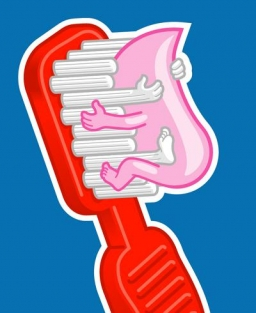# Examination 7504

7. Class B is having a preventive dental check-up. Two dental caries were found in a quarter of the pupils and one caries in an eighth. Half of the class had all their teeth healthy. How many pupils go to 7th B if three pupils are absent on the day of the dental examination?

n =  24

### Step-by-step explanation:

n/4 + n/8 + n/2 + 3 = n

n = 24

n = 24/1 = 24

n = 24

Our simple equation calculator calculates it.Did you find an error or inaccuracy? Feel free to write us. Thank you!

Tips for related online calculators
Need help calculating sum, simplifying, or multiplying fractions? Try our fraction calculator.
Do you have a linear equation or system of equations and looking for its solution? Or do you have a quadratic equation?